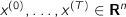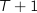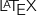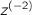# CVXGEN: Code Generation for Convex Optimization

## Indexed parameters and variables

Many optimization problems contain a sequence of variables or parameters, indexed by time or some other offset. For example, in a model predictive control (MPC) problem, the state variables are typically. Rather than declare allvariables individually, CVXGEN provides special syntax to work with indexed variables. Here are some examples:

parameters
A[i] (m,n) nonnegative, i=1..3
end

variables
x[t] (n), t=0..T
z[tau], tau=-L..L
end

In CVXGEN'soutput, these will be indexed with a superscript, bracketed index, such as.

Indexed symbols can be used in sum functions like sum[t=0..T](x[t]), or in indexed constraints.